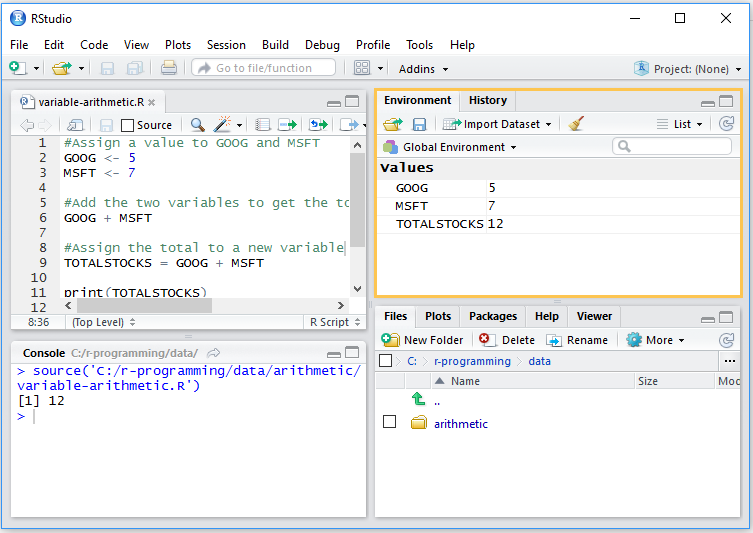# Using Variables in R

In R programming, we will be using variables all the time, so it is important to understand what a variable is and how it works in R.

A variable refers to a named storage that allows us to store data which our R programs can refer to and manipulate. A variable in R can store a value or an object (more on objects later). For example, we can store value ’50’ in a variable called ‘x’. This is called variable assignment, that is, the variable ‘x’ is assigned value ’50’.

The variables can be assigned values using leftward, rightward and equal to operator, however, the most common way is to use the leftward operator ( <- )

Once we have assigned a value to a variable, we can print it by just calling the variable name or by using the print() or the cat() function.

# Assignment using equal operator.
x = 50

# Assignment using leftward operator.
y <- "table"

# Assignment using rightward operator.
100 -> z

x

print (x)
cat ("x is ", x ,"\n")
cat ("y is ", y ,"\n")
cat ("z is ", z ,"\n")


When executed, this will produce the following result:

 50
x is 50
y is table
z is 100


In RStudio, there are various ways you can execute the R script.

• Select a code line and Click the ‘Run’ button in the script window. This will execute that line in the code. Select multiple lines of code and click the ‘Run’ button in the script window. This will execute all the selected lines in the code.
• To execute the entire script (using the Source command), press CTRL+SHIFT+ENTER. This will execute the entire script with each source command printed in the console. This is called Source with Echo.
• You can also press CTRL+SHIFT+S to run the Source command, but without Echo. This means that only the output will be printed to console, not the source commands.

### Arithmetic Operations Using Variables

Once you have assigned variables, you can use these variables to perform arithmetic operations. Let’s say you have 5 Google stocks in your stock portfolio and 7 Microsoft stocks.

In R, you will create variables for these stocks and assign values to them. After that you can calculate the total stocks in portfolio by performing arithmetic operations on these variables and assign the total value to a new variable.

#Assign a value to GOOG and MSFT
GOOG <- 5
MSFT <- 7

#Add the two variables to get the total stocks in the portfolio
GOOG + MSFT

#Assign the total to a new variable
TOTALSTOCKS = GOOG + MSFT
print (TOTALSTOCKS)


Try running this script in RStudio. Also try to run it using different commands described above and notice the difference in the output:

• Select all lines of code and press CTRL + ENTER (Or press the R button) to execute all lines of the code.
• Press CTRL + SHIFT + ENTER to execute the script using the Source command with each source command printed in the console (Source with Echo).
• Press CTRL + SHIFT + S to execute the script using the Source command with each source command without echoing the commands in the console.

### Change in Environment

As you assign the variables, you will also notice one more important change. As we learned earlier, all the objects you create in your current R session will be added to the environment. In this case, you will see three variables in the environment as shown below:RStudio Environment Variables

### Variable types

r is smart enough to understand the type of data you’re assigning to the variables. In the above examples, the values assigned were numeric and R could interpret them as numeric therefore allowed us to add the values in the two variables. But what will happen if one variable has a numeric value and the other has a character value and you try adding the two? In such a situation, r will throw an error and will ask you to provide numeric data type for the binary operation.

To test this, try running the following code in RStudio:

#Assign a value to GOOG and MSFT
GOOG <- 5
MSFT <- 'stock'

#Add the two variables to get the total stocks in the portfolio
GOOG + MSFT